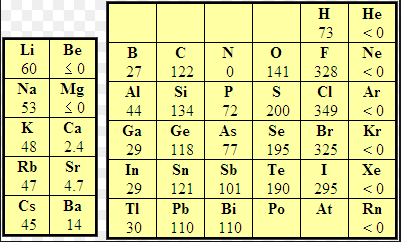Electron affinity (EA) is defined as the energy exchanged (usually released) when an isolated ground-state gas atom picks up an electron to form an anion. Thus the F atom releases 328 kJ/mol when it captures an electron and becomes $F^-$. The fluoride anion is very stable because it has the electron configuration of neon.

\begin{equation} F(g)+1e^-\rightarrow F^-(g) \end{equation}

Although the atoms that release the most energy when capturing an electron are found on the periodic table on the right, it is observed that metals such as lithium, in a gaseous state, also release energy by forming lithium anions.

\begin{equation} Li(g) + e^-\rightarrow Li^-(g) \end{equation}

The heat released is: AE=-59.6 kJ/mol.Some elements such as alkaline earths (group 2) do not show a tendency to capture electrons because they have a complete 2s subshell. The nitrogen group does not have a tendency to take electrons either, since it would mean starting to compete with the np subshell.

Some elements can take more than one electron, but energy is only released on the first capture, the rest are endothermic.

In general, the electron affinity increases to the left and upwards, with the exceptions of group 2 and 15. Another exception to this rule should be noted, which we find in the halogen group: chlorine has a higher electron affinity than fluorine. Due to its small size, fluorine presents important electronic repulsions that make it difficult to capture the electron, thus explaining a lower electron affinity value than chlorine.